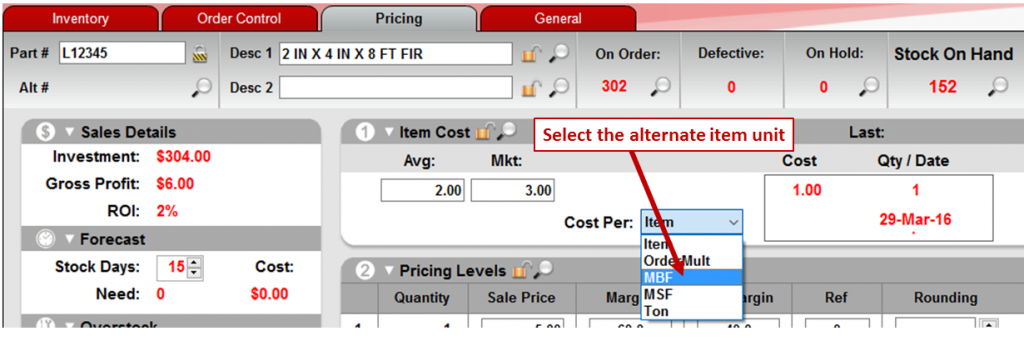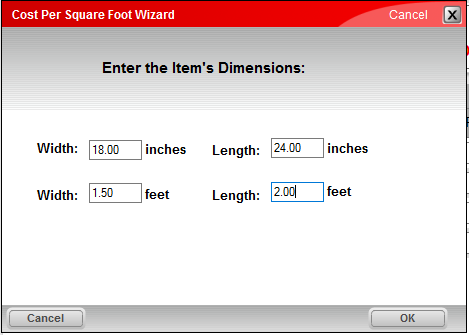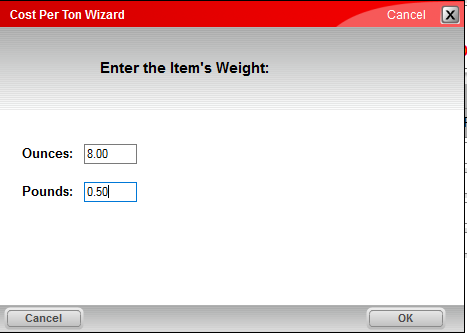You can view item costs in different units, such as thousand board feet (MBF) and tons, in both the Inventory and PO modules. This feature is especially useful for products that are priced by suppliers in a different unit of measure than you use for your inventory items. Building materials and feed/grain mixtures are common examples. For example, lumber is traditionally priced by the supplier in units of a thousand board feet, and sold as individual pieces. Similarly, a feed mixture may be purchased by the ton, but sold in small quantities by weight.

With this feature, you can view item cost in the following ways:

• Cost per thousand board feet (MBF) based on the item’s thickness, width and length.
• Cost per thousand square feet (MSF) based on the item’s width and length.
• Cost per ton based on the item’s weight in pounds or ounces.
• Cost per minimum order quantity. This option does not involve any unit conversion.

The following example shows how this feature works.

## Lumber (MBF)

Let’s assume you sell lumber with the following dimensions, 2 inches x 4 inches x 8 feet, and you want to see the item cost in MBF (thousand board feet) units.

To do this, in the Inventory module, open the lumber item, and then click the Pricing tab.

In the Item Cost pane, in the Cost Per list, select MBF.When you select MBF, a Cost of Lumber Wizard appears. Enter the dimensions for this lumber item, and then click OK.After the wizard closes, new PO numbers appear in the Item Cost pane. These numbers show the item costs for a thousand board feet (MBF).In this example, the average cost (Avg) of \$2 is used to calculate the first PO value of \$375 per MBF. Similarly, the market value cost (Mkt) of \$3 is used to calculate the second PO value of \$562.50 per MBF. The Last box displays the item cost of \$1.00 from the most recent purchase order as well as the equivalent MBF cost of \$187.50.

Note: To ensure precise PO cost values, calculated numbers are saved at much higher accuracy (more values after the decimal point) than we display.

To save the MBF calculations, click F12 Save.

Once the MBF costs are saved with the inventory item, both the cost for the item, and the equivalent MBF cost, will appear when you add this item to a purchase order.## MSF and Ton

You can use the same process that we used in the MBF example to compute costs for thousand square foot (MSF) and Ton units.

Cost Per Square Foot Wizard

To use Cost Per Square Foot Wizard, enter a value for each dimension—width and length—in either inches or feet. Values entered in inches are also automatically shown in feet. Similarly, values entered in feet are converted to inches. For example, if you enter a width of 1.5 feet, the wizard also displays the width as 18 inches.Cost Per Ton Wizard

To use the Cost Per Ton Wizard, enter a value in either ounces or pounds. When you enter one of these weight measures, the other is calculated automatically. For example, if you enter 8 ounces, the wizard also displays the equivalent value of 0.5 pounds.## Item and OrderMult

Item and OrderMult are additional options, but do not do any unit conversion.

The Item option is selected by default, and does not perform any additional calculations. With this option, the information in the Item Cost pane is the same as before.

If you select the OrderMult (stands for order multiple) option, the PO values give you the purchase order cost for the item’s order quantity. The order quantity is found in the Order Qty box on the Order Control tab. In the example below, the order quantity is 100, each item cost is \$2, and the calculated PO costs are all \$200.Note: The PO value for the OrderMult option is informational only. Unlike the MBF, MSF and Ton options, this PO value does not appear when you add the item to a purchase order.